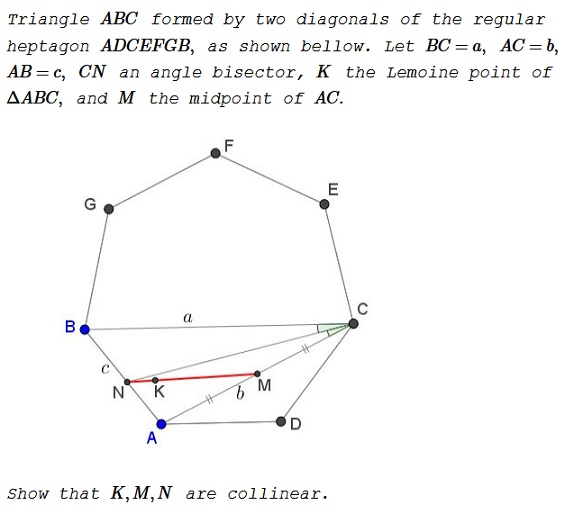# Problem 4096 from Crux Mathematicorum

### Problem### Solution

In barycentric coordinates, relative to $\Delta ABC,\,$ $N=(a:b:0),\,$ $K=(a^2:b^2:c^2),\,$ $M=(1:0:1).\,$ For the three points to be collinear it is necessary and sufficient to have

$\left|\begin{array}{ccc}a&a^2&1\\b&b^2&0\\0&c^2&1\end{array}\right|=0,$

which is equivalent to

(1)

$c^2+ab=a^2.$

Consider the cyclic quadrilateral $ABEC.\,$Since, $ADCEFB\,$ is a regular heptagon, $CE=AB=c\,$ and $EB=EA=BC=a.\,$ Applying Ptolemy's theorem to quadrilateral $ABEC\,$ we have

$AB\cdot CE+AC\cdot BE=BC\cdot EA.$

which is $c\cdot c+b\cdot a=a\cdot a,\,$ i.e., (1). Thus the three points are indeed collinear.

### Acknowledgment

This is Problem 4096 from Crux Mathematicorum, V. 42, NO. 10 December 2016. The problem is by Kadir Altintas. The solution is by Michel Bataille and Titu Zvonaru.